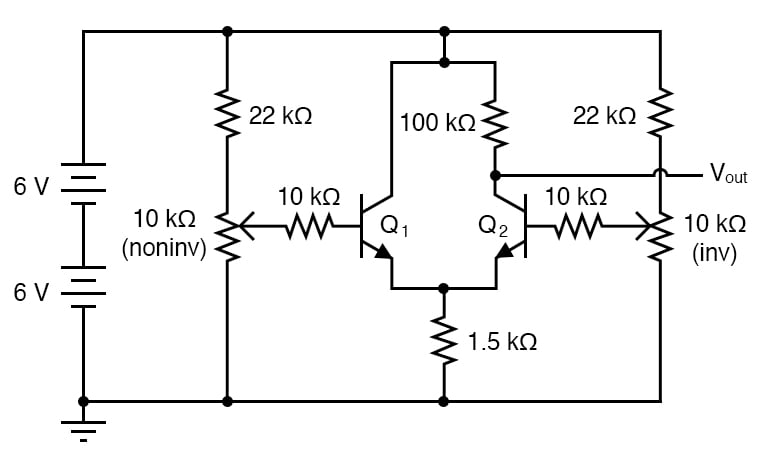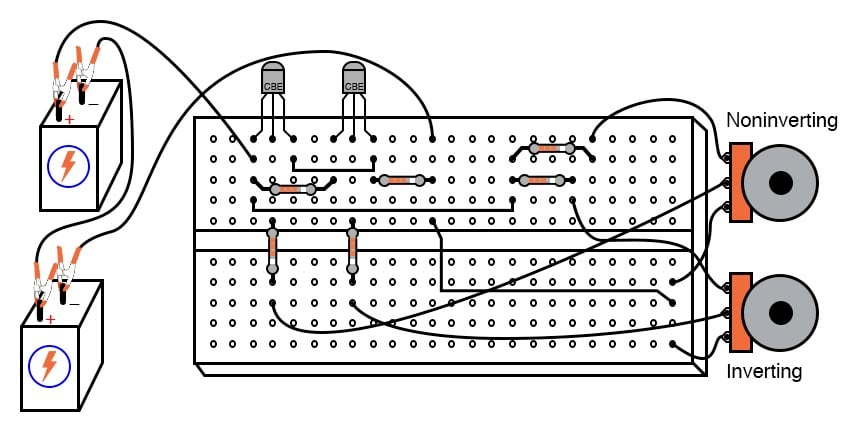# Differential Amplifier

## Chapter 5 - Discrete Semiconductor Circuits

PARTS AND MATERIALS

• Two 6-volt batteries
• Two NPN transistors—models 2N2222 or 2N3403 recommended (Radio Shack catalog # 276-1617 is a package of fifteen NPN transistors ideal for this and other experiments)
• Two 10 kΩ potentiometers, single-turn, linear taper (Radio Shack catalog # 271-1715)
• Two 22 kΩ resistors
• Two 10 kΩ resistors
• One 100 kΩ resistor
• One 1.5 kΩ resistor

Resistor values are not especially critical in this experiment, but have been chosen to provide high voltage gain for a “comparator-like” differential amplifier behavior.

CROSS-REFERENCES

Lessons In Electric Circuits, Volume 3, chapter 4: “Bipolar Junction TransistorsLessons In Electric Circuits, Volume 3, chapter 8: “Operational Amplifiers

LEARNING OBJECTIVES

SCHEMATIC DIAGRAMILLUSTRATIONINSTRUCTIONS

This circuit forms the heart of most operational amplifier circuits: the differential pair. In the form shown here, it is a rather crude differential amplifier, quite nonlinear and unsymmetrical with regard to output voltage versus input voltage(s).

With a high voltage gain created by a large collector/emitter resistor ratio (100 kΩ/1.5 kΩ), though, it acts primarily as a comparator: the output voltage rapidly changing value as the two input voltage signals approach equality. Measure the output voltage (voltage at the collector of Q2 with respect to ground) as the input voltages are varied.

Note how the two potentiometers have different effects on the output voltage: one input tends to drive the output voltage in the same direction (noninverting), while the other tends to drive the output voltage in the opposite direction (inverting). This is the essential nature of a differential amplifier: two complementary inputs, with contrary effects on the output signal.

Ideally, the output voltage of such an amplifier is strictly a function of the difference between the two input signals. This circuit falls considerably short of the ideal, as even a cursory test will reveal.

An ideal differential amplifier ignores all common-mode voltage, which is whatever level of voltage common to both inputs. For example, if the inverting input is at 3 volts and the noninverting input at 2.5 volts, the differential voltage will be 0.5 volts (3 - 2.5) but the common-mode voltage will be 2.5 volts since that is the lowest input signal level.

Ideally, this condition should produce the same output signal voltage as if the inputs were set at 3.5 and 3 volts, respectively (0.5 volts differential, with a 3-volt common-mode voltage). However, this circuit does not give the same result for the two different input signal scenarios. In other words, its output voltage depends on both the differential voltage and the common-mode voltage.

As imperfect as this differential amplifier is, its behavior could be worse. Note how the input signal potentiometers have been limited by 22 kΩ resistors to an adjustable range of approximately 0 to 4 volts, given a power supply voltage of 12 volts.

If you’d like to see how this circuit behaves without any input signal limiting, just bypass the 22 kΩ resistors with jumper wires, allowing full 0 to 12-volt adjustment range from each potentiometer. Do not worry about building up excessive heat while adjusting potentiometers in this circuit!

Unlike the current mirror circuit, this circuit is protected from thermal runaway by the emitter resistor (1.5 kΩ), which doesn’t allow enough transistor current to cause any problem.

RELATED WORKSHEET:

• Share
Published under the terms and conditions of the Design Science License